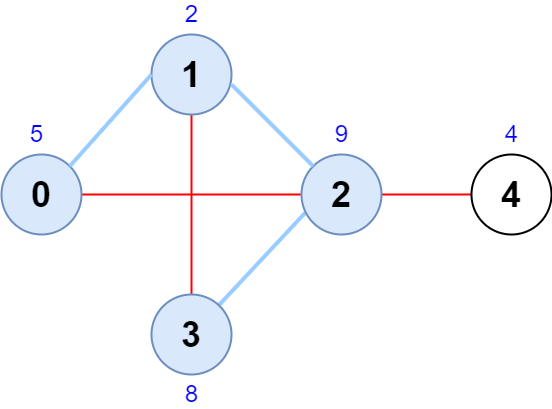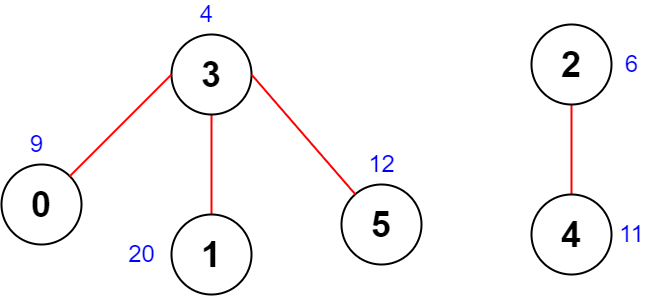##### Welcome to Subscribe On Youtube

Formatted question description: https://leetcode.ca/all/2242.html

# 2242. Maximum Score of a Node Sequence

• Difficulty: Hard.
• Related Topics: Array, Graph, Sorting, Enumeration.
• Similar Questions: Get the Maximum Score.

## Problem

There is an undirected graph with n nodes, numbered from 0 to n - 1.

You are given a 0-indexed integer array scores of length n where scores[i] denotes the score of node i. You are also given a 2D integer array edges where edges[i] = [ai, bi] denotes that there exists an undirected edge connecting nodes ai and bi.

A node sequence is valid if it meets the following conditions:

• There is an edge connecting every pair of adjacent nodes in the sequence.

• No node appears more than once in the sequence.

The score of a node sequence is defined as the sum of the scores of the nodes in the sequence.

Return the **maximum score of a valid node sequence with a length of 4. If no such sequence exists, return **-1.

Example 1:Input: scores = [5,2,9,8,4], edges = [[0,1],[1,2],[2,3],[0,2],[1,3],[2,4]]
Output: 24
Explanation: The figure above shows the graph and the chosen node sequence [0,1,2,3].
The score of the node sequence is 5 + 2 + 9 + 8 = 24.
It can be shown that no other node sequence has a score of more than 24.
Note that the sequences [3,1,2,0] and [1,0,2,3] are also valid and have a score of 24.
The sequence [0,3,2,4] is not valid since no edge connects nodes 0 and 3.


Example 2:Input: scores = [9,20,6,4,11,12], edges = [[0,3],[5,3],[2,4],[1,3]]
Output: -1
Explanation: The figure above shows the graph.
There are no valid node sequences of length 4, so we return -1.


Constraints:

• n == scores.length

• 4 <= n <= 5 * 104

• 1 <= scores[i] <= 108

• 0 <= edges.length <= 5 * 104

• edges[i].length == 2

• 0 <= ai, bi <= n - 1

• ai != bi

• There are no duplicate edges.

## Solution

• class Solution {
public int maximumScore(int[] scores, int[][] edges) {
// store only top 3 nodes (having highest scores)
int[][] graph = new int[scores.length];
for (int[] a : graph) {
Arrays.fill(a, -1);
}
for (int[] edge : edges) {
insert(edge, graph[edge], scores);
insert(edge, graph[edge], scores);
}
int maxScore = -1;
for (int[] edge : edges) {
int u = edge;
int v = edge;
int score = scores[u] + scores[v];
for (int i = 0; i < 3; i++) {
// if neighbour is current node, skip
if (graph[u][i] == -1 || graph[u][i] == v) {
continue;
}
for (int j = 0; j < 3; j++) {
// if neighbour is current node or already choosen node, skip
if (graph[v][j] == -1 || graph[v][j] == u) {
continue;
}
if (graph[v][j] == graph[u][i]) {
continue;
}
maxScore =
Math.max(maxScore, score + scores[graph[u][i]] + scores[graph[v][j]]);
}
}
}
return maxScore;
}

private void insert(int n, int[] arr, int[] scores) {
if (arr == -1) {
arr = n;
} else if (arr == -1) {
if (scores[arr] < scores[n]) {
arr = arr;
arr = n;
} else {
arr = n;
}
} else if (arr == -1) {
if (scores[arr] < scores[n]) {
arr = arr;
arr = arr;
arr = n;
} else if (scores[arr] < scores[n]) {
arr = arr;
arr = n;
} else {
arr = n;
}
} else {
if (scores[arr] < scores[n]) {
arr = arr;
arr = n;
} else if (scores[arr] < scores[n]) {
arr = n;
}
}
}
}

############

class Solution {
public int maximumScore(int[] scores, int[][] edges) {
int n = scores.length;
List<Integer>[] g = new List[n];
Arrays.setAll(g, k -> new ArrayList<>());
for (int[] e : edges) {
int a = e, b = e;
}
for (int i = 0; i < n; ++i) {
g[i].sort((a, b) -> scores[b] - scores[a]);
g[i] = g[i].subList(0, Math.min(3, g[i].size()));
}
int ans = -1;
for (int[] e : edges) {
int a = e, b = e;
for (int c : g[a]) {
for (int d : g[b]) {
if (c != b && c != d && a != d) {
int t = scores[a] + scores[b] + scores[c] + scores[d];
ans = Math.max(ans, t);
}
}
}
}
return ans;
}
}

• class Solution:
def maximumScore(self, scores: List[int], edges: List[List[int]]) -> int:
g = defaultdict(list)
for a, b in edges:
g[a].append(b)
g[b].append(a)
for k in g.keys():
g[k] = nlargest(3, g[k], key=lambda x: scores[x])
ans = -1
for a, b in edges:
for c in g[a]:
for d in g[b]:
if b != c != d != a:
t = scores[a] + scores[b] + scores[c] + scores[d]
ans = max(ans, t)
return ans

############

# 2242. Maximum Score of a Node Sequence
# https://leetcode.com/problems/maximum-score-of-a-node-sequence/

class Solution:
def maximumScore(self, scores: List[int], edges: List[List[int]]) -> int:
d = defaultdict(list)

def go(x, y):
if len(d[x]) == 3:
heapq.heappushpop(d[x], (scores[y], y))
else:
heapq.heappush(d[x], (scores[y], y))

for x, y in edges:
go(x, y)
go(y, x)

res = float('-inf')

for x, y in edges:
for score1, node1 in d[x]:
for score2, node2 in d[y]:
if node1 not in (x, y) and node2 not in (x, y) and node1 != node2:
res = max(res, scores[x] + scores[y] + score1 + score2)

return -1 if res == float('-inf') else res



Explain:

nope.

Complexity:

• Time complexity : O(n).
• Space complexity : O(n).